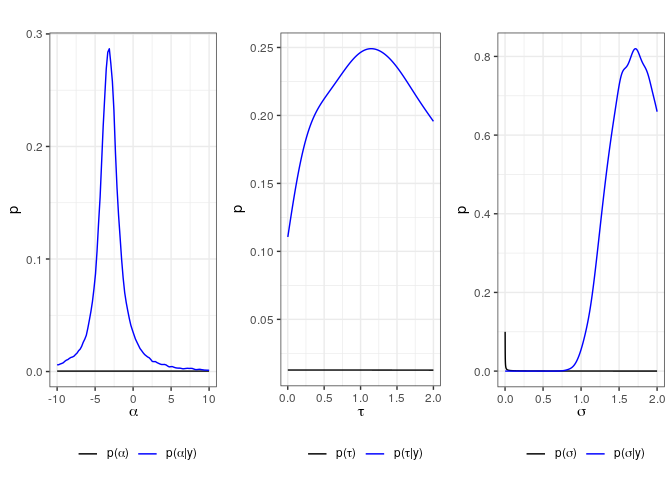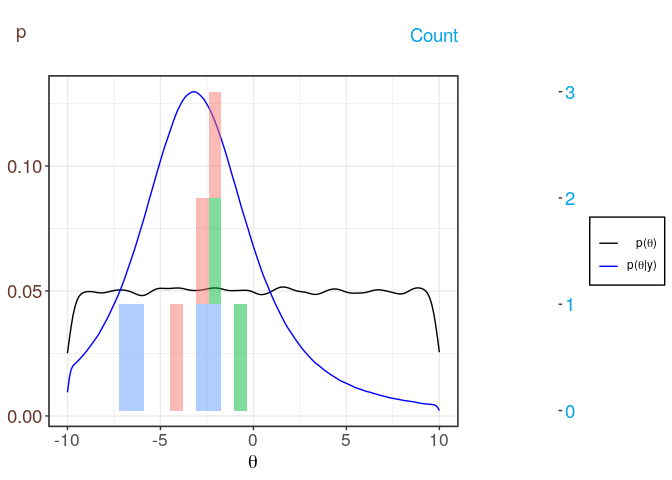# Using exPrior by virtue of a simple example

When performing hydro-geological investigation at a given site, measurements at that site $$y^*$$ are often used to characterize the parameter $$\theta$$ of a given model. By using the information from the previously studied sites, we wish to derive a prior distribution for a parameter $$\theta$$. The exPrior package allows a user to compute informative prior distribution(s) for a parameter $$\theta$$ using such external data.

For simplicity, this vignette only demonstrates the package’s application in a three level hierarchical Bayesian model, which is:

• Level 1: Data $$y$$ relating to $$\theta$$ from the sites.
• Level 2: These data $$y_{i,j}$$ are a realization of a Gaussian distribution with site specific, local means $$\mu_i$$ and global variance $$\sigma^2$$, i.e $$y_{i,j} \sim N(\mu_i, \sigma^2)$$
• Level 3: The site specific means $$\mu_j$$ are a again realization of a Gaussian distribution with parameters $$\alpha$$ and $$\tau$$, defining a common prior pdf of the hyperparameter $$\eta = (\alpha, \tau, \sigma)$$.

With this set up, consider an example where data are available at 3 sites:

• Site 1: $$y_{1,1} = -2,\ y_{1,2} = -3,\ y_{1,2} = -4$$
• Site 2: $$y_{2,1} = -2,\ y_{2,2} = -1$$
• Site 3: $$y_{3,1} = -6,\ y_{3,2} = -7,\ y_{3,3} = -2,\ y_{3,4} = -3$$

Using these data, we want to get the prior distribution of $$\theta$$. We’ll solve this task by using the function genExPrior. This function will perform two steps:

1. Given data from a number of sites, the function calculates the posterior distribution $$p(\eta|y)$$ for the hyperparameters $$\eta$$ of our statistical model. A Markov Chain Monte-Carlo (MCMC) method is employed to compute these posterior distributions.

2. From these posterior hyperparameter distribution, this package derives the prior of $$\theta$$ at new site $$S_0$$, i.e. $$p(\theta|y)$$.

library(devtools)
## Loading required package: usethis
load_all()
## Warning: 1 components of ... were not used.
##
## We detected these problematic arguments:
## * action
##
## Did you misspecify an argument?
## Loading exPrior
## Loading required package: nimble
## nimble version 0.8.0 is loaded.
## please visit http://R-nimble.org.
##
## Attaching package: 'nimble'
## The following object is masked from 'package:stats':
##
##     simulate
##
## Attaching package: 'testthat'
## The following object is masked from 'package:devtools':
##
##     test_file
library(exPrior)

Under the assumption that the site specific parameter follows a normal distribution, the function genExPrior takes in three parameters. First, exdata is a data frame where the first column contains the data and the second column is a site index where the data come from. Second, $\theta$ is a vector of numerical values where to evaluate the prior distribution. Finally, niter is an integer for the sample size in the MCMC that is used to evaluate unknown $$\mu_i, \sigma^2_j$$ at each site i ( i = 1, 2, 3 in our case). By default it is set to $$10^5$$, which is an effective sample size for MCMC. Users are free to choose a different sample size.

Putting the data of the three sites into dataframe, we have:

exdata = data.frame(val = c(c(-2,-3,-4), c(-2,-1), c(-6,-7,-2,-3)),
site_id = c(rep('S1',3), rep('S2',2), rep('S3',4)))
exdata
##   val site_id
## 1  -2      S1
## 2  -3      S1
## 3  -4      S1
## 4  -2      S2
## 5  -1      S2
## 6  -6      S3
## 7  -7      S3
## 8  -2      S3
## 9  -3      S3
theta = seq(from=-10, to=10, by=0.1)

Running genExPrior with these arguments, we get the prior distribution for $$\theta$$ as well as the posterior hyperparameters of our Bayesian hierarchical model.

  resExPrior = genExPrior(exdata = exdata, theta = theta)
## defining model...
## building model...
## setting data and initial values...
## running calculate on model (any error reports that follow may simply reflect missing values in model variables) ...
## checking model sizes and dimensions... This model is not fully initialized. This is not an error. To see which variables are not initialized, use model\$initializeInfo(). For more information on model initialization, see help(modelInitialization).
## model building finished.
## compiling... this may take a minute. Use 'showCompilerOutput = TRUE' to see C++ compilation details.
## compilation finished.
##  conjugate_dnorm_dnorm sampler: alpha
##  RW sampler: chSqTau
##  RW sampler: sigma
##  RW sampler: xiTau_negOrPos
##  conjugate_dnorm_dnorm sampler: mu
##  conjugate_dnorm_dnorm sampler: mu
##  conjugate_dnorm_dnorm sampler: mu
## thin = 1: alpha, chSqTau, sigma, xiTau_negOrPos, tau
## compiling... this may take a minute. Use 'showCompilerOutput = TRUE' to see C++ compilation details.
## compilation finished.
## |-------------|-------------|-------------|-------------|
## |-------------------------------------------------------|

If the distribution of the parameter is not normal, genExPrior provides an option to transform the distribution to normal under user’s choices. Two types of Johnson transformation, logarithm and log ratio, as well as Box-Cox transformation are provided. Lower and upper limit of log ratio, and value of $$\lambda$$ for Box-Cox transformation should be chosen so that the transformed data has normal distribution.

First, let us look at the posteriors of the hyperparameters, which are conditioned on the data in the exdata data frame. To that end, we use the function plotHyperDist with the results from genExPrior as input

    plotHyperDist(resExPrior)Then, we can visualize both the uninformative and informative distribution of $$\theta$$ using plotExPrior. This function again takes as input the output from genExPrior as well as a Boolean asking whether to additionally plot the used data.

    plotExPrior(resExPrior, plotExData = TRUE)
## stat_bin() using bins = 30. Pick better value with binwidth.## NULL